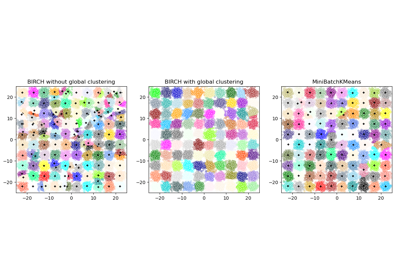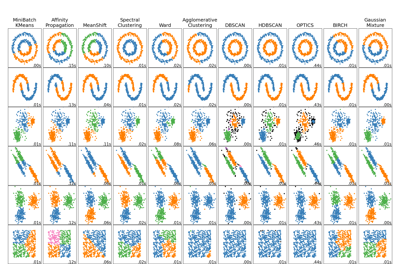# sklearn.cluster.Birch¶

class sklearn.cluster.Birch(*, threshold=0.5, branching_factor=50, n_clusters=3, compute_labels=True, copy=True)[source]

Implements the BIRCH clustering algorithm.

It is a memory-efficient, online-learning algorithm provided as an alternative to MiniBatchKMeans. It constructs a tree data structure with the cluster centroids being read off the leaf. These can be either the final cluster centroids or can be provided as input to another clustering algorithm such as AgglomerativeClustering.

Read more in the User Guide.

New in version 0.16.

Parameters:
thresholdfloat, default=0.5

The radius of the subcluster obtained by merging a new sample and the closest subcluster should be lesser than the threshold. Otherwise a new subcluster is started. Setting this value to be very low promotes splitting and vice-versa.

branching_factorint, default=50

Maximum number of CF subclusters in each node. If a new samples enters such that the number of subclusters exceed the branching_factor then that node is split into two nodes with the subclusters redistributed in each. The parent subcluster of that node is removed and two new subclusters are added as parents of the 2 split nodes.

n_clustersint, instance of sklearn.cluster model, default=3

Number of clusters after the final clustering step, which treats the subclusters from the leaves as new samples.

• None : the final clustering step is not performed and the subclusters are returned as they are.

• sklearn.cluster Estimator : If a model is provided, the model is fit treating the subclusters as new samples and the initial data is mapped to the label of the closest subcluster.

• int : the model fit is AgglomerativeClustering with n_clusters set to be equal to the int.

compute_labelsbool, default=True

Whether or not to compute labels for each fit.

copybool, default=True

Whether or not to make a copy of the given data. If set to False, the initial data will be overwritten.

Attributes:
root__CFNode

Root of the CFTree.

dummy_leaf__CFNode

Start pointer to all the leaves.

subcluster_centers_ndarray

Centroids of all subclusters read directly from the leaves.

subcluster_labels_ndarray

Labels assigned to the centroids of the subclusters after they are clustered globally.

labels_ndarray of shape (n_samples,)

Array of labels assigned to the input data. if partial_fit is used instead of fit, they are assigned to the last batch of data.

n_features_in_int

Number of features seen during fit.

New in version 0.24.

feature_names_in_ndarray of shape (n_features_in_,)

Names of features seen during fit. Defined only when X has feature names that are all strings.

New in version 1.0.

MiniBatchKMeans

Alternative implementation that does incremental updates of the centers’ positions using mini-batches.

Notes

The tree data structure consists of nodes with each node consisting of a number of subclusters. The maximum number of subclusters in a node is determined by the branching factor. Each subcluster maintains a linear sum, squared sum and the number of samples in that subcluster. In addition, each subcluster can also have a node as its child, if the subcluster is not a member of a leaf node.

For a new point entering the root, it is merged with the subcluster closest to it and the linear sum, squared sum and the number of samples of that subcluster are updated. This is done recursively till the properties of the leaf node are updated.

References

Examples

>>> from sklearn.cluster import Birch
>>> X = [[0, 1], [0.3, 1], [-0.3, 1], [0, -1], [0.3, -1], [-0.3, -1]]
>>> brc = Birch(n_clusters=None)
>>> brc.fit(X)
Birch(n_clusters=None)
>>> brc.predict(X)
array([0, 0, 0, 1, 1, 1])


Methods

 fit(X[, y]) Build a CF Tree for the input data. fit_predict(X[, y]) Perform clustering on X and returns cluster labels. fit_transform(X[, y]) Fit to data, then transform it. get_feature_names_out([input_features]) Get output feature names for transformation. get_params([deep]) Get parameters for this estimator. partial_fit([X, y]) Online learning. Predict data using the centroids_ of subclusters. set_params(**params) Set the parameters of this estimator. Transform X into subcluster centroids dimension.
fit(X, y=None)[source]

Build a CF Tree for the input data.

Parameters:
X{array-like, sparse matrix} of shape (n_samples, n_features)

Input data.

yIgnored

Not used, present here for API consistency by convention.

Returns:
self

Fitted estimator.

property fit_

DEPRECATED: fit_ is deprecated in 1.0 and will be removed in 1.2.

fit_predict(X, y=None)[source]

Perform clustering on X and returns cluster labels.

Parameters:
Xarray-like of shape (n_samples, n_features)

Input data.

yIgnored

Not used, present for API consistency by convention.

Returns:
labelsndarray of shape (n_samples,), dtype=np.int64

Cluster labels.

fit_transform(X, y=None, **fit_params)[source]

Fit to data, then transform it.

Fits transformer to X and y with optional parameters fit_params and returns a transformed version of X.

Parameters:
Xarray-like of shape (n_samples, n_features)

Input samples.

yarray-like of shape (n_samples,) or (n_samples, n_outputs), default=None

Target values (None for unsupervised transformations).

**fit_paramsdict

Returns:
X_newndarray array of shape (n_samples, n_features_new)

Transformed array.

get_feature_names_out(input_features=None)[source]

Get output feature names for transformation.

Parameters:
input_featuresarray-like of str or None, default=None

Only used to validate feature names with the names seen in fit.

Returns:
feature_names_outndarray of str objects

Transformed feature names.

get_params(deep=True)[source]

Get parameters for this estimator.

Parameters:
deepbool, default=True

If True, will return the parameters for this estimator and contained subobjects that are estimators.

Returns:
paramsdict

Parameter names mapped to their values.

partial_fit(X=None, y=None)[source]

Online learning. Prevents rebuilding of CFTree from scratch.

Parameters:
X{array-like, sparse matrix} of shape (n_samples, n_features), default=None

Input data. If X is not provided, only the global clustering step is done.

yIgnored

Not used, present here for API consistency by convention.

Returns:
self

Fitted estimator.

property partial_fit_

DEPRECATED: partial_fit_ is deprecated in 1.0 and will be removed in 1.2.

predict(X)[source]

Predict data using the centroids_ of subclusters.

Avoid computation of the row norms of X.

Parameters:
X{array-like, sparse matrix} of shape (n_samples, n_features)

Input data.

Returns:
labelsndarray of shape(n_samples,)

Labelled data.

set_params(**params)[source]

Set the parameters of this estimator.

The method works on simple estimators as well as on nested objects (such as Pipeline). The latter have parameters of the form <component>__<parameter> so that it’s possible to update each component of a nested object.

Parameters:
**paramsdict

Estimator parameters.

Returns:
selfestimator instance

Estimator instance.

transform(X)[source]

Transform X into subcluster centroids dimension.

Each dimension represents the distance from the sample point to each cluster centroid.

Parameters:
X{array-like, sparse matrix} of shape (n_samples, n_features)

Input data.

Returns:
X_trans{array-like, sparse matrix} of shape (n_samples, n_clusters)

Transformed data.

## Examples using sklearn.cluster.Birch¶Compare BIRCH and MiniBatchKMeans

Compare BIRCH and MiniBatchKMeansComparing different clustering algorithms on toy datasets

Comparing different clustering algorithms on toy datasets